CAT  >  Practice Questions Level 1: Functions - 1

# Practice Questions Level 1: Functions - 1 - Notes | Study Level-wise Practice Questions for CAT Preparation - CAT

 1 Crore+ students have signed up on EduRev. Have you?

This EduRev document offers 10 Multiple Choice Questions (MCQs) from the topic Functions (Level - 1). These questions are of Level - 1 difficulty and will assist you in the preparation of CAT & other MBA exams. You can practice/attempt these CAT Multiple Choice Questions (MCQs) and check the explanations for a better understanding of the topic.

Question for Practice Questions Level 1: Functions - 1
Try yourself:If f(x) = y and g(x) = y2 + 1, find g(f(x)).

Question for Practice Questions Level 1: Functions - 1
Try yourself:If ,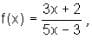then

Question for Practice Questions Level 1: Functions - 1
Try yourself:The graph of the function y = 5x6 + 3x4 - x2 + 8

Question for Practice Questions Level 1: Functions - 1
Try yourself:If f(x, y) = 3x2 - 2xy - y2 + 4, find f(1, -1).

Question for Practice Questions Level 1: Functions - 1
Try yourself:The given graph is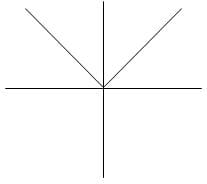Question for Practice Questions Level 1: Functions - 1
Try yourself:A function is defined as f(x) =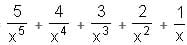+ 1 + x + 2x2 + 3x3 + 4x4 + 5x5. If f(2) = 260.78, what is the value of f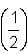?

Question for Practice Questions Level 1: Functions - 1
Try yourself:Find the range of the function f(x) =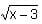Question for Practice Questions Level 1: Functions - 1
Try yourself:f : → R and g : R → R are defined as f (x) = x2 and g (x) = x + 3, x ∈ R, then (fog) (x) = ?

Question for Practice Questions Level 1: Functions - 1
Try yourself:Let a function be fn+1(x) = fn (x) + 3 . If f2(2) = 4, find the value of f6(2).

Question for Practice Questions Level 1: Functions - 1
Try yourself:Let A = {a, b, c}. Then, the range of the relation R = {(a, b), (a, c), (b, c)} defined on A is

The document Practice Questions Level 1: Functions - 1 - Notes | Study Level-wise Practice Questions for CAT Preparation - CAT is a part of the CAT Course Level-wise Practice Questions for CAT Preparation.
All you need of CAT at this link: CAT

## Level-wise Practice Questions for CAT Preparation

277 docs
 Use Code STAYHOME200 and get INR 200 additional OFF

## Level-wise Practice Questions for CAT Preparation

277 docs

### How to Prepare for CAT

Read our guide to prepare for CAT which is created by Toppers & the best Teachers

Track your progress, build streaks, highlight & save important lessons and more!

,

,

,

,

,

,

,

,

,

,

,

,

,

,

,

,

,

,

,

,

,

;